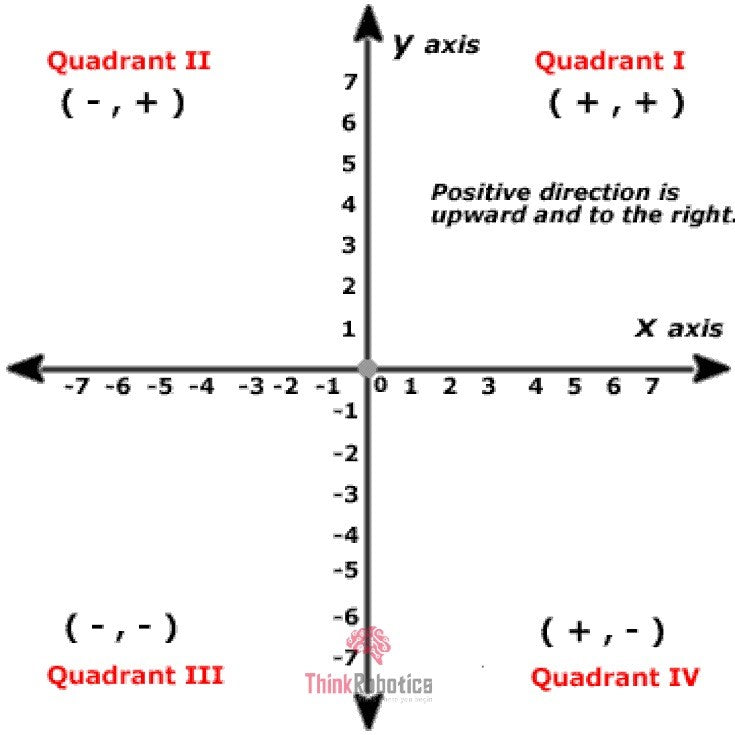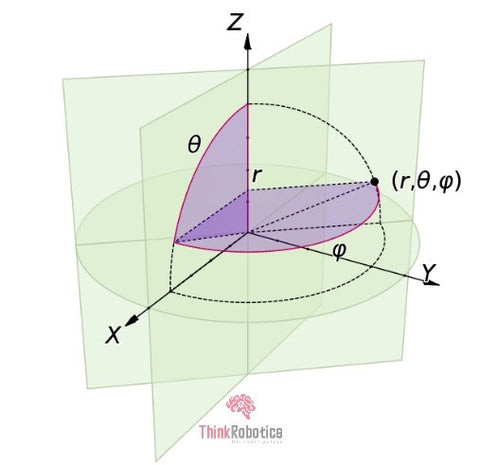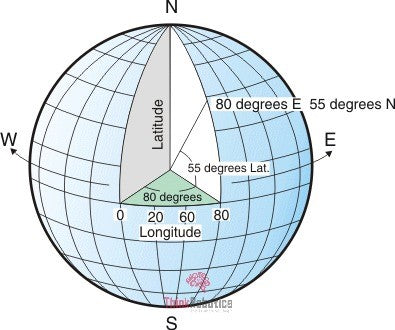Free Shipping for orders over ₹1999

support@thinkrobotics.com | +91 93183 94903# Coordinate System | ThinkRobotics.in

Generally, a coordinate system is a system that uses one or more numbers, or coordinates, to locate the position of any point uniquely, line, place, or object concerning some reference system.

For example, the location of any place is obtained by placing its coordination on a map. That is what the global positioning system does since it is impossible to have a precisely defined address for each and everything in the world.

Types of Coordinate System:

The Coordinate system is a fundamental idea of defining the location in any system, and thus this system is altered according to the needs of the field. Here are a few prominent ones.

#### Cartesian Coordinate System:

The simplest one is a Cartesian coordinate system, named for the 17th-century mathematician and philosopher René Descartes. A Cartesian coordinate system is simply a grid formed by placing two measurement scales or axes, one horizontal (X-axis) and one vertical (Y-axis). The point at which both X and Y values are zero is called the coordinate system's origin.Fig 1: A  2-D cartesian coordinate system

This is the case with the 2-Dimensional system. When higher dimensions are used, a greater number of scales came into the picture. Below is an example of  3 -Dimensional and 4- Dimensional Cartesian coordinate system.Fig 2: A 4-D Cartesian and 3-D cartesian system

#### Polar Coordinate System:

A polar coordinate system is a two-dimensional coordinate system. Each point on a plane is determined by a distance from a reference point and an angle from a reference direction. The polar coordinate system is an alternate coordinate system where the two variables are r and θ, instead of X and Y.

The polar grid is denoted as a series of concentric circles radiating out from the pole. A distance measured from the pole is called Radius (r). A ray from the pole in the reference direction is called the Polar axis. The reference point of the polar graph is known as Pole.

An angle measured from the polar axis, usually counter-clockwise, is called Angular coordinate (θ).  This is only a 2-D system because we have only two coordinates.Fig 3: A Polar coordinate system

#### Cylindrical and Spherical Coordinate System:

The polar coordinate system is extended, resulting in the Cylindrical and the Spherical Coordinate system.

The Cylindrical-coordinate system involves an additional Z-axis as the one in the 3-D cartesian system. Giving coordinates as (r , θ, Z ).Fig 4: A Cylindrical Coordinate system

The Spherical coordinate system is a 3-D extension of the Polar Coordinate system, which uses two angle coordinates (θφ) and one distance coordinate (ρ or r) to locate a point, that is (ρθφ) coordinates.Fig 5: A Spherical coordinate system

These are a few of the very important and widely used coordinate systems. The systems mentioned above are used in mathematics, Physics, and mostly for research purposes a few more are mentioned below.

#### Curvilinear Coordinate System:

Here, the coordinate lines may be curved. These coordinates can be derived from a set of Cartesian coordinates using a locally invertible transformation (a one-to-one map) at each point.

#### Orthogonal Coordinate System:

Coordinates here are defined as a set of d coordinates q = (q1, q2, ..., QD) in which the coordinate surfaces all meet at right angles.

#### Skew Coordinate System:

A skew coordinate system is a curvilinear coordinate system where the coordinate surfaces are not orthogonal, in contrast to orthogonal coordinates.

#### Log-polar Coordinate System:

This system represents a point in the plane by the logarithm of the distance from the origin and an angle measured from a reference line intersecting the origin.

#### Barycentric Coordinate System:

It is a coordinate system in which a point's location is specified by reference to a simplex (a triangle for points in a plane, a tetrahedron for points in three-dimensional space, etc.). The barycentric coordinates of a point can be interpreted as masses placed at the simplex vertices, such that the point is the center of mass (or barycentre) of these masses.

#### Right-handed Coordinate System:

Here, the direction in which your hand closes to make a fist in the direction of a positive rotation around an axis signified by the extended right-hand thumb.

#### Left-handed Coordinate System:

Here, the direction in which your hand closes to make a fist in the direction of a positive rotation around an axis signified by the extended left-hand thumb.

Other than the systems mentioned above, a coordinate system is used to locate points on Earth.

#### Geographic Coordinate System:

A geographic coordinate system is a 3-dimensional reference system that locates points on the Earth's surface. The unit of measure is usually decimal degrees. A point has two coordinates: latitude and longitude. Latitude and longitude measure angles.

Latitude is defined as the angle formed by the intersection of a line perpendicular to the Earth's surface at a point and the Equator's plane. Latitude values range from -90 to +90 degrees.

A meridian, or line of longitude, is formed by a plane that passes through the point and the North and South poles.Fig 6: A geographic Coordinate system

A geographic coordinate system has the following components:

• Angular units: The unit of measure on the spherical reference system.
• Spheroid: The reference spheroid for the coordinate transformation.
• Datum: Defines the relationship of the reference spheroid to the Earth's surface.
• Prime meridian: The longitude origin of the spherical reference system.

#### Usage in day to day life:

• You use the geographic coordinates provided by GPS to specify a location precisely.
• The chess notation (e.g., e2-e4) uses a coordinate system to describe the moves succinctly and clearly. Even the grid-based games follow the same.
• The coordinate systems are exceptionally vital for programming (basically anything which manipulates images, including drawing tables on the screen) or solving mathematical/geometry problems.
• With the help of coordinate geometry, we can create mathematical models of objects or things. Using different mathematical tools, such as geometric transformations, can move them, change their orientation, enlarge, or shrink them.
• The Cartesian coordinate system is generally the standard system used in most Computer Aided Drawing (CAD) programs. Generally, used to obtain Solid & Surface Modelling, assembling them, Drafting Details and even Reverse Engineering.
• A map projection transforms the Earth's surface into a plane. This is done with the help of the Geographic Coordinate system.
• The 3D positioning system of a 3D printer corresponds to the overall operation of its x, y, and z-axes of the cartesian coordinate system.
• The x and y axes correspond to the 3D printer's lateral movement, and the z-axis corresponds to vertical movement.
• Air traffic is handled and regulated by using coordinate geometry. Coordinates of any aircraft are used to describe its current location. Even if an aircraft moves a small distance (up, down, forward, or backward), its coordinates are updated in the system, which is in turn controlled by the air traffic controllers.
• Coordinate geometry deals with lines and graphs profit/loss, bar graphs, pie charts, etc. It can have 2D graphs in general, and sometimes 3D graphs. It brings out a connection between algebra and geometry through graphs of lines and curves.
• In economics, the Lorenz Curve is the graphical representation of the cumulative distribution function of the empirical probability distribution of wealth or income. Here, again we used a coordinate system.
• The Cartesian coordinate system is highly used in military services in determining the position of the targets.
• The State Plane Coordinate systems of all 50 states of the USA (starting with North Carolina in the 1930s) is based on coordinate geometry.

Sources: